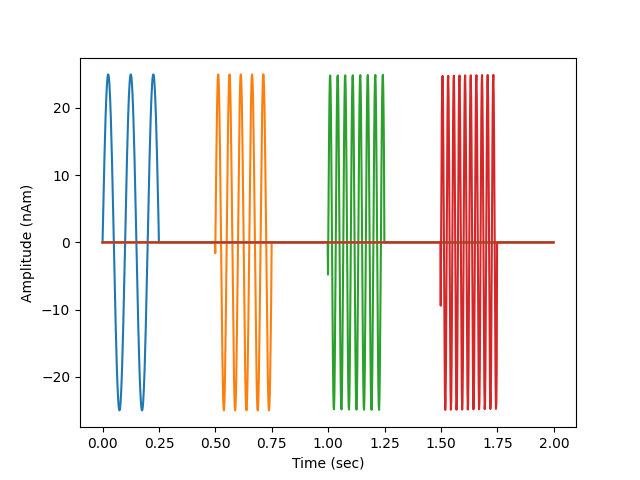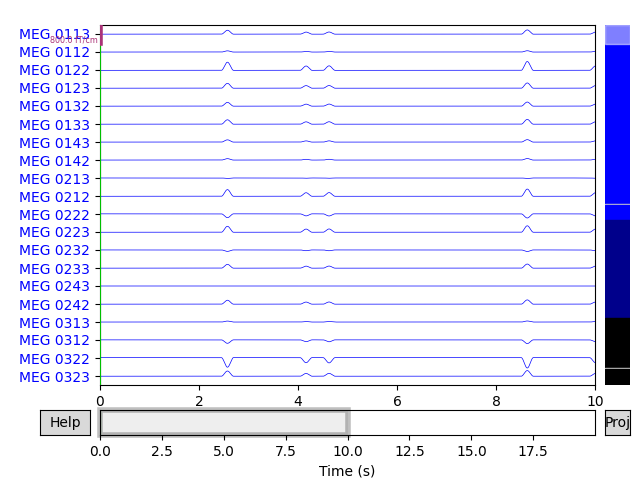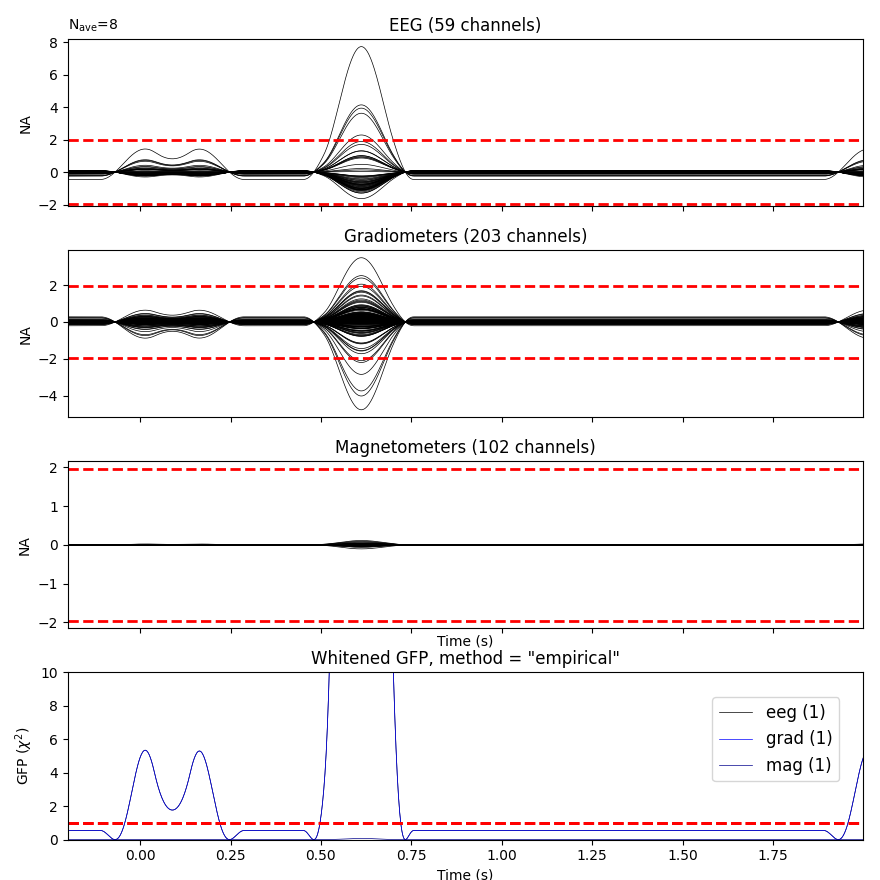# Generate simulated raw data¶

This example generates raw data by repeating a desired source activation multiple times.

# Authors: Yousra Bekhti <yousra.bekhti@gmail.com>
#          Mark Wronkiewicz <wronk.mark@gmail.com>
#          Eric Larson <larson.eric.d@gmail.com>
#

import numpy as np
import matplotlib.pyplot as plt

import mne
from mne import find_events, Epochs, compute_covariance, make_ad_hoc_cov
from mne.datasets import sample
from mne.simulation import (simulate_sparse_stc, simulate_raw,

print(__doc__)

data_path = sample.data_path()
raw_fname = data_path + '/MEG/sample/sample_audvis_raw.fif'
fwd_fname = data_path + '/MEG/sample/sample_audvis-meg-eeg-oct-6-fwd.fif'

# Load real data as the template
raw.set_eeg_reference(projection=True)


Out:

Opening raw data file /home/circleci/mne_data/MNE-sample-data/MEG/sample/sample_audvis_raw.fif...
Read a total of 3 projection items:
PCA-v1 (1 x 102)  idle
PCA-v2 (1 x 102)  idle
PCA-v3 (1 x 102)  idle
Range : 25800 ... 192599 =     42.956 ...   320.670 secs
1 projection items deactivated


Generate dipole time series

n_dipoles = 4  # number of dipoles to create
epoch_duration = 2.  # duration of each epoch/event
n = 0  # harmonic number
rng = np.random.RandomState(0)  # random state (make reproducible)

def data_fun(times):
"""Generate time-staggered sinusoids at harmonics of 10Hz"""
global n
n_samp = len(times)
window = np.zeros(n_samp)
start, stop = [int(ii * float(n_samp) / (2 * n_dipoles))
for ii in (2 * n, 2 * n + 1)]
window[start:stop] = 1.
n += 1
data = 25e-9 * np.sin(2. * np.pi * 10. * n * times)
data *= window
return data

times = raw.times[:int(raw.info['sfreq'] * epoch_duration)]
src = fwd['src']
stc = simulate_sparse_stc(src, n_dipoles=n_dipoles, times=times,
data_fun=data_fun, random_state=rng)
# look at our source data
fig, ax = plt.subplots(1)
ax.plot(times, 1e9 * stc.data.T)
ax.set(ylabel='Amplitude (nAm)', xlabel='Time (sec)')
mne.viz.utils.plt_show()Out:

Reading forward solution from /home/circleci/mne_data/MNE-sample-data/MEG/sample/sample_audvis-meg-eeg-oct-6-fwd.fif...
Computing patch statistics...
[done]
Computing patch statistics...
[done]
Desired named matrix (kind = 3523) not available
Read MEG forward solution (7498 sources, 306 channels, free orientations)
Desired named matrix (kind = 3523) not available
Read EEG forward solution (7498 sources, 60 channels, free orientations)
MEG and EEG forward solutions combined
Source spaces transformed to the forward solution coordinate frame


Simulate raw data

raw_sim = simulate_raw(raw.info, [stc] * 10, forward=fwd, verbose=True)
add_noise(raw_sim, cov, iir_filter=[0.2, -0.2, 0.04], random_state=rng)
raw_sim.plot()Out:

Setting up raw simulation: 1 position, "cos2" interpolation
Event information stored on channel:              STI 014
Interval 0.000-2.000 sec
Setting up forward solutions
Computing gain matrix for transform #1/1
Interval 0.000-2.000 sec
Interval 0.000-2.000 sec
Interval 0.000-2.000 sec
Interval 0.000-2.000 sec
Interval 0.000-2.000 sec
Interval 0.000-2.000 sec
Interval 0.000-2.000 sec
Interval 0.000-2.000 sec
Interval 0.000-2.000 sec
10 STC iterations provided
Done
Adding noise to 366/376 channels (366 channels in cov)
Sphere                : origin at (0.0 0.0 0.0) mm
Source location file  : dict()
Assuming input in millimeters
Assuming input in MRI coordinates

Positions (in meters) and orientations
1 sources
ecg simulated and trace not stored
Setting up forward solutions
Computing gain matrix for transform #1/1
Sphere                : origin at (0.0 0.0 0.0) mm
Source location file  : dict()
Assuming input in millimeters
Assuming input in MRI coordinates

Positions (in meters) and orientations
2 sources
blink simulated and trace stored on channel:      EOG 061
Setting up forward solutions
Computing gain matrix for transform #1/1


Plot evoked data

events = find_events(raw_sim)  # only 1 pos, so event number == 1
epochs = Epochs(raw_sim, events, 1, tmin=-0.2, tmax=epoch_duration)
cov = compute_covariance(epochs, tmax=0., method='empirical',
verbose='error')  # quick calc
evoked = epochs.average()
evoked.plot_white(cov, time_unit='s')Out:

Trigger channel has a non-zero initial value of 1 (consider using initial_event=True to detect this event)
Removing orphaned offset at the beginning of the file.
9 events found
Event IDs: 
9 matching events found
Applying baseline correction (mode: mean)
Created an SSP operator (subspace dimension = 4)
4 projection items activated
Computing rank from covariance with rank=None
Using tolerance 1.1e-14 (2.2e-16 eps * 59 dim * 0.84  max singular value)
Estimated rank (eeg): 1
EEG: rank 1 computed from 59 data channels with 1 projector
Computing rank from covariance with rank=None
Using tolerance 1.8e-13 (2.2e-16 eps * 203 dim * 3.9  max singular value)
GRAD: rank 1 computed from 203 data channels with 0 projectors
Computing rank from covariance with rank=None
Using tolerance 4.3e-15 (2.2e-16 eps * 102 dim * 0.19  max singular value)
Estimated rank (mag): 1
MAG: rank 1 computed from 102 data channels with 3 projectors
Created an SSP operator (subspace dimension = 4)
Computing rank from covariance with rank={'eeg': 1, 'grad': 1, 'mag': 1, 'meg': 2}
Setting small MEG eigenvalues to zero (without PCA)
Setting small EEG eigenvalues to zero (without PCA)
Created the whitener using a noise covariance matrix with rank 3 (361 small eigenvalues omitted)


Total running time of the script: ( 0 minutes 14.261 seconds)

Estimated memory usage: 157 MB

Gallery generated by Sphinx-Gallery